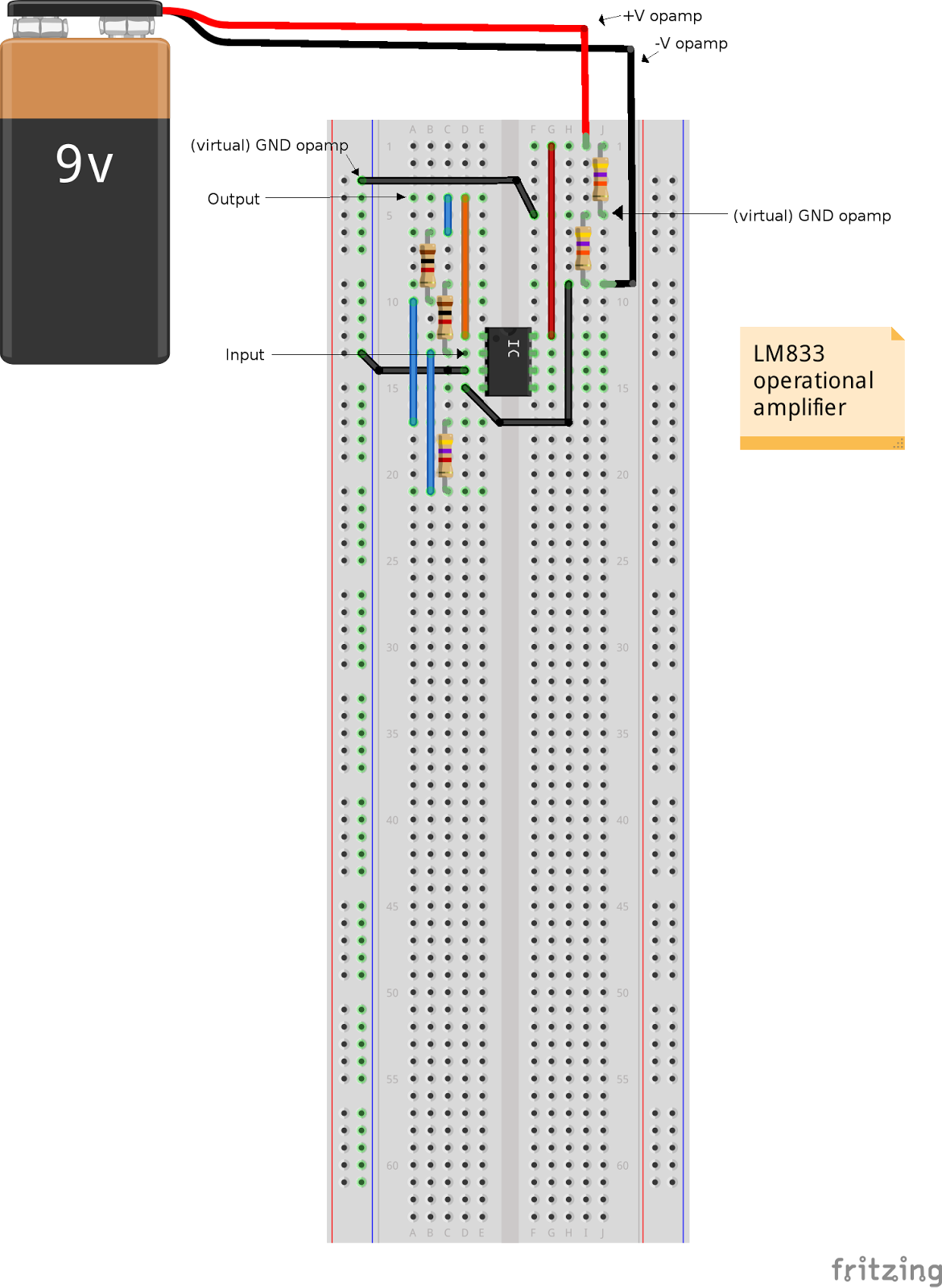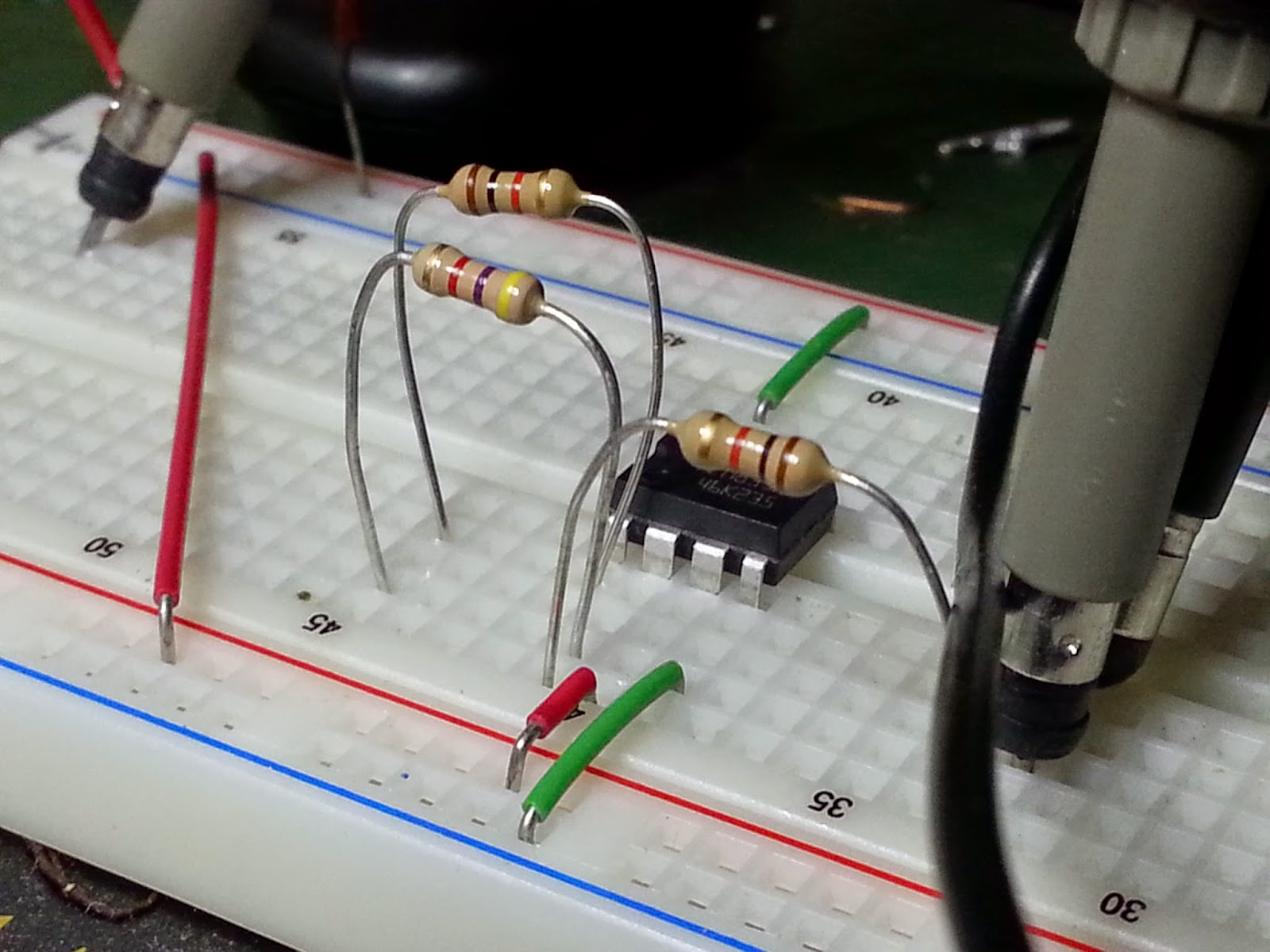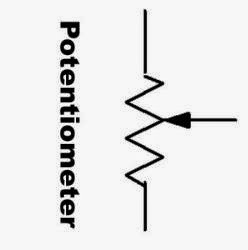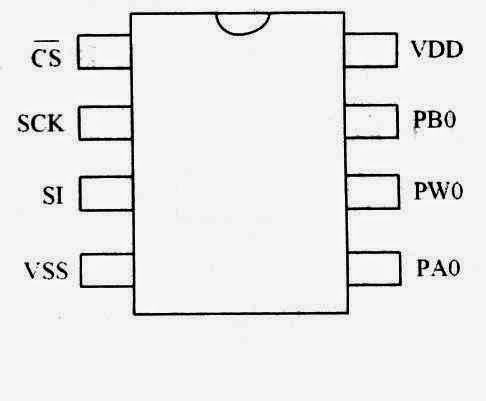## sabato 7 marzo 2015

### How to control an inverting amplifier using Arduino and a digital potentiometer.

An inverting amplifier is a differential amplifier in which that circuit's non-inverting input is grounded, and inverting input is identified with Vinput.An inverting amplifier

The closed-loop gain is R2 / R1, hence Voutput = - R2/R1 Vinput so Voutput/Vinput = G = -R2/R1 and GdB = 20 log(|-R2/R1|) = 20 log(R2/R1). For example, if R1 and R2 where 1kohm and 10kohm, G = 10 V/V and GdB = 20 dB, if R1 and R2 where 2kohm and 15kohm, G = 7.5 V/V and GdB = 17.5 dB. (Inverting amplifier).

If you want to simulate a circuit, you can use the well-known software LTspice. This is what we will do with our amplifier.
First we draw the schematic and then we perform PSPICE schematics circuit simulation (in the time domain and in the frequency domain).An inverting amplifier under test on LTSpice

We want to determine the gain of the amplifier depending on the value of R2 resistor.
In this schematic there is a SPICE directive (.step param R 1k 10k 1k) which determines what resistance to start at (R2 = R), what resistance to stop at (10kohm) , and how much will it increment (1kohm). If we simulate (in the frequency domain) we can see that the GdB value changes according to the R2 value:

R1 = 1KOhm, R2 = 1KOhm, |G| = 1, GdB = 0 dB, Vin-peak = 0.1 V, Vout-peak = 0.1 V
R1 = 1KOhm, R2 = 2KOhm, |G| = 2, GdB = 6 dB, Vin-peak = 0.1 V, Vout-peak = 0.2 V
R1 = 1KOhm, R2 = 3KOhm, |G| = 3, GdB = 9.54 dB, Vin-peak = 0.1 V, Vout-peak = 0.3 V
R1 = 1KOhm, R2 = 4KOhm, |G| = 4, GdB = 12 dB, Vin-peak = 0.1 V, Vout-peak = 0.4 V
R1 = 1KOhm, R2 = 5KOhm, |G| = 5, GdB = 14 dB, Vin-peak = 0.1 V, Vout-peak = 0.5 V
R1 = 1KOhm, R2 = 6KOhm, |G| = 6, GdB = 15.6 dB, Vin-peak = 0.1 V, Vout-peak = 0.6 V
R1 = 1KOhm, R2 = 7KOhm, |G| = 7, GdB = 16.9 dB, Vin-peak = 0.1 V, Vout-peak = 0.7 V
R1 = 1KOhm, R2 = 8KOhm, |G| = 8, GdB = 18 dB, Vin-peak = 0.1 V, Vout-peak = 0.8 V
R1 = 1KOhm, R2 = 9KOhm, |G| = 9, GdB = 19.1 dB, Vin-peak = 0.1 V, Vout-peak = 0.9 V
R1 = 1KOhm, R2 = 10KOhm, |G| = 10, GdB = 20 dB, Vin-peak = 0.1 V, Vout-peak = 1 VSome bode diagrams show the gain changing according to the R2 value

In the picture below you can see the variations of Voutput according to the G value (depending on R2 value)The amplitude of the output signal changes according to the G value

Now we can make a simple prototype with fixed gain. If we choose R1 and R2 equal to 1 kOhm, Rd equal to 4.7 kOhm the gain is G = - (R2 + Rd)/R1 = 5.7 V/V (15.12 dB).Inverting amplifier with fixed gainThe wiring scheme of the inverting amplifier with fixed gainThe inverting amplifier under test

The picture below shows the input (with peak value of 0.42V) and the output signals (with peak value of 2.32V), so the absolute value of the gain is 2.32V/0.42V = 5.52 V/V. You can see from the picture that they are exact opposites (180 degrees out of phase) because this is an inverting amplifier.The values of the input and output signals

Now it's  time to put a digital potentiometer instead of the Rd resistor. For further informations about the digital potentiometer controlled by Arduino, have a look to the article "How to control a digital potentiometer using Arduino UNO"

Connect your circuit as shown in the picture below:The inverting amplifier with the digital potentiometer controlled by Arduino UNO

Download the sketch from our github repository. For the first test we set a Rd value equal to 4.7 kohm so in the sketch the "loop" function should be like that:

void loop() {
int i = 128;                                 // R = 4700 ohm
spi_out(CS_signal, cmd_byte2, i);
}

Load the sketch on Arduino, put a sinusoidal signal in input (amplitude 0.4 - 0.5 V, f = 1 khz) and measure the output.
In my test circuit I have this situation: input signal max peak 0.4V and f = 1 khz, output max peak 2.24V, so the gain is 5.6The values of the input and output signals (digital potentiometer)

Now we change dinamically the gain of the amplifier, switching between two values of Rd (4.7 kohm and 9 khom) so in the sketch the "loop" function should be like that:

void loop() {
int i = 128;                                                                // R = 4700 ohm
spi_out(CS_signal, cmd_byte2, i);
Serial.print("value i = ");
Serial.print(i);
Serial.println("   R = 4700 ohm");
delay(5000);

i = 0;                                                                      // R = 9000 ohm
spi_out(CS_signal, cmd_byte2, i);
Serial.print("value i = ");
Serial.print(i);
Serial.println("     R = 9000 ohm");
delay(5000);
}

In the clip below you can see the result of this test:

The gain switches between 5.4 (corresponding to a Rd = 4.7 kohm) and 9 (corresponding to a Rd = 9 kohm).

In the video below I show the value of Rd measured using a digital multimeter (without the op amp)

## sabato 28 febbraio 2015

### How to control a digital potentiometer using Arduino UNO

A potentiometer is a 3-terminal device used as a variable resistor. It has a rotating contact used as a voltage divider (see on Wikipedia).A classical potentiometerThe symbol of the potentiometer

A voltage divider is a simple circuit where the output voltage is a fraction of the input voltage. You can use two fixed resistor or a potentiometer.A simple example of voltage divider with a potentiometer

These examples are about mechanical potentiometers but there are types of potentiometers which are completely electronic and adjustable using proper input signals, they are called digital potentiometers

Today I want to test one of them, a MPC41010, which is a single potentiometer. Its max value is 10kohm (min value is not exactly 0 ohm but 100ohm).A MCP41010 chipA pinout diagram of a MCP41010

The name of the chip depends on the max value of the potentiometer and the number of them inside the chip:
MPC41010: single potentiometer, 10 khom
MPC41050: single potentiometer, 50 khom
MPC41100: single potentiometer, 100 khom
MCP42010: two independent potentiometers, 10 khom
MCP42050: two independent potentiometers, 50 khom
MCP42100: two independent potentiometers, 100 khom

To drive it I use an Arduino UNO board and its SPI port.
If you read their datasheet,you see that to program these chips you have first to send a "command byte" (to tell the chip what to do) and a "data byte" (to tell the chip which value of resistance to set, from 0 to 255). For example, to set a resistance value at 10kohm, you have to set the data byte to 11111111 (255), to set the value at 5kohm, you have to set the data byte to 10000000 (128).From the datasheet: "Executing any command is accomplished by setting CS low and then clocking-in a command byte followed by a data byte into the 16-bit shift register. The command is executed when CS is raised."

Connect your test circuit as showed in the picture below:Circuit schematic (Fritzing)

In the sketch, there are two loops: the first one gradually increase the resistance on the LED, the second one gradually decrease the resistance on the LED. The result is that the LED glows (as you can see in the video below).

1 - http://nicksen782.blogspot.it/2010/06/digital-potentiometers-mcp42010.html

2 -  http://www.frankensteingarage.it/blog/2012/07/04/potenziometri-digitali/ (in Italian)

## mercoledì 5 marzo 2014

### How to make a bluetooth datalogger for temperature and humidity.

In this project I use an Arduino Mega (2560) board, a DHT22 sensor (more accurate than DHT11 sensor), a 10 kΩ resistor, a 10 kΩ trimmer, a 2 x 16 LCD display, a HC-05 bluetooth serial adapter, a RTC module (from Adafruit: http://www.adafruit.com/products/264) and a SD card module (from Adafruit: http://www.adafruit.com/products/254).

This is a complete datalogger for temperature and humidity, infact it saves these two values with the time every 10 minutes (you can set this frequency in the sketch as you like, even if I suggest not less than 10 minutes because the file becomes too big) in a CSV file stored into the SD card. The sketch creates automatically the path for the log files using the path /templog/yy/mm/dd. You can read the logs putting the SD card into your pc's card reader or using the bluetooth connection.

Here are the sketch, the python script and the Fritzing schematic.Schematic (Fritzing, available on the repository)

After connecting the parts, create a folder in the SD card and name it templog.Power on your Arduino. It will soon start to measure temperature and humidity and to save them on the SD card.Example of Bluetooth module with adapter

Inside the sketch you can set some variables:

max_battery_voltage        if the power supply is a battery you can set here its voltage (the display shows the charge level)

minutes_updatefile        the sketch updates the log file every "minutes_updatefile" minutes

seconds_updatedatehour        the sketch updates the date and the time on the lcd display every "seconds_updatedatehour" seconds

seconds_updatemeasurelcd        the sketch updates the temperature and the humidity values on the lcd display every "seconds_updatemeasurelcd" seconds

seconds_updatemeasurebattery        the sketch updates the measure of the charge of the battery every "seconds_updatemeasurebattery" seconds

pathbase        this is the root folder for the logs on the SD card (default is templog/)

The sketch has some internal commands:

rf&:   the "read file" command
sf&:   the "size file" command
mth&:  the "measure t/h" command
rdt&:  the "read date/time" command
tsd&:  the "type sd card" command
ssd&:  the "size sd card" command
fm&:   the "free memory size" command
sdt&:  the "update date and time" command
rbt&:  the "read voltage battery" command

You can interact with the data logger via the bluetooth connection and the script test.py. Download this script from the github repository and put the name and the MAC address of your bluetooth module in this script (for example, in my script they are BT1 and 00:13:04:10:06:20).

Here are some examples of usage:

python test.py -dn BT1 -cl "mth&?" reads temperature and humidity
python test.py -dn BT1 -cl "rdt&?" reads date and hour
python test.py -dn BT1 -cl "sf&14/01/20?" reads the size of the specified file  (format:  yy/mm/dd)
python test.py -dn BT1 -cl "rf&14/01/20?" reads the specified file  (format:  yy/mm/dd)
python test.py -dn BT1 -cl "tsd&?" reads the type of SD card
python test.py -dn BT1 -cl "ssd&?" reads the size of SD card
python test.py -dn BT1 -cl "fm&?" reads the size of free memory in microcontroller
python test.py -dn BT1 -cl "sdt&2014&01&21&14&30&00?" sets date and hour (format:  yyyy, mm, dd, hh, mm, ss)
python test.py -dn BT1 -cl "rbt&?" reads battery voltageThe datalogger workingA zoom of the display

## domenica 9 giugno 2013

### How to make a wifi datalogger for temperature and humidity.

In this project I use two Arduino boards: the first one (Arduino UNO) is used for measuring temperature and humidity, the second one (Arduino MEGA 2560) for wifi connection
to my home lan. The two boards communicate each other through serial connection.

The Arduino UNO board has a DHT11 sensor (but you can use a more accurate DHT22 sensor) with a 10 kΩ resistor, a RTC module (from Adafruit: http://www.adafruit.com/products/264) and
a SD card module (from Adafruit: http://www.adafruit.com/products/254). This is a complete datalogger for temperature and humidity, infact it saves these two values with the time every 10 minutes (you can set this frequency in the sketch as you like, even if I suggest not less than 10 minutes because the file becomes too big) in a CSV file stored into the SD card. The sketch creates automatically the path for the log files using the path /templog/yy/mm/dd. You can read the logs putting the SD card into your pc's card reader.
The sketch provides some APIs for serial communication. The commands are:
1) sf& (size file):  the syntax is sf&yy/mm/dd?
If you type this command on the serial monitor, the board will show the size of the file /templog/yy/mm/dd
2) rf& (read file): the syntax is rf&yy/mm/dd&startbyte&stopbyte?
If you type this command on the serial monitor, the board will read the file /templog/yy/mm/dd FROM "startbyte" TO "stopbyte" and will send it through the serial port.
So, if you want read the whole file, you must put startbyte = 0 and stopbyte = size of the file (in bytes). This syntax allows to split the file in several chunks, if it  is too big.

For example: the log for April 24th 2013 is in the file /templog/13/04/24, suppose its size is 2184 bytes. First you want to know the size of the file so you must type the command sf&13/04/24? on the serial monitor. The board will reply printing the value 2184. Then you can read the whole file typing the command rf&13/04/24&0&2184?

So:
1 - connect the Arduino UNO board as shown in the figure below
2 - create the "templog" folder in the SD card
3 - insert the SD card in its module
4 - download the sketch test_comunicazione_seriale_UNO.ino from   https://github.com/rickit69/techrm/tree/master/test_comunicazione_seriale_UNO
7 - load the sketch

The board will start to save temperature, humidity, hour, minutes every 10 minutes. Use the serial monitor as explained above, to interrogate the board.Wiring scheme

You can use the second board (Arduino MEGA 2560 with Weburban wifi module) to download the log files wireless:
1 - wire the board following the instructions in the article http://techrm.blogspot.it/2012/12/how-to-interface-arduino-mega-2560-to.html  and download the modified wireless     library from the same article
2 - download the sketch test_comunicazione_seriale_MEGA.ino from   https://github.com/rickit69/techrm/tree/master/test_comunicazione_seriale_MEGA

The sketch communicates serially to the UNO board through the Serial3 port. So wire the two boards following the scheme below:

MEGA                   UNO
pin 14         to         pin 0
pin 15         to         pin 1
GND          to         GND

Now open a shell and go in the folder where you downloaded the python script. These are the parameters for the script:

[techrm@notebook-dell test_comunicazione_seriale_python]\$ python test.py -h
usage: test.py [-h] [-c COMMAND] [-y YEAR] [-m MONTH] [-d DAY] [-v]

optional arguments:
-h, --help            show this help message and exit
-c COMMAND, --command COMMAND
the command
-y YEAR, --year YEAR  year (yy)
-m MONTH, --month MONTH
month (mm)
-d DAY, --day DAY     day (dd)
-v                    verbose

In particular, -c is the parameter for the command. In the script the only command is "rf" (read file). For example, we want to download the log file for May 06th 2013 , so type
in the shell the command line   python test.py -c rf -y 13 -m 05 -d 06
If you want to redirect the output to a file, type the command line   python test.py -c rf -y 13 -m 05 -d 06 > 13_05_06.txt

Finally, I wish to thanks my friend Mauro Pala for his suggestions about C language.

## domenica 24 marzo 2013

### How to remotely measure a temperature using Arduino and WiFi

First you need an Arduino Mega 2560 board and a Weburban WiFi board (this board uses standard connectors over SPI to an Arduino compatible Microchip 802.11b/g/n MRF24WB0MA).
Connect them as showed in http://techrm.blogspot.it/2012/12/how-to-interface-arduino-mega-2560-to.html
In this example I use ArduinoIDE version 1.0.3.
Follow the instructions about the setting of the WiShield library (you can load it from https://github.com/rickit69/techrm/tree/master/WiShield_Arduino_IDE_1.0.3). This library is modified to properly work with Arduino Mega 2560 board AND ArduinoIDE version 1.0.3.

Now you need a DS18S20 digital thermometer (http://www.maximintegrated.com/datasheet/index.mvp/id/2815) and a 4.7 kΩ resistor. Now connect them to the Arduino board as shown in the picture:

Now you need to download two applications and a library from https://github.com/rickit69/techrm:
1) test_wifi_temperature, a simple sketch for measure temperature remotely
2) test_arduino_temp_wifi, a simple php application to use with test_wifi_temperature sketch

Place the OneWire folder into your Arduino Library. Here are the default library folder locations:
On  Mac:: In (home directory)/Documents/Arduino/libraries
On  Windows:: My Documents -> Arduino -> libraries
On  Linux:: (home directory)/sketchbook/libraries

Put the folder test_arduino_temp_wifi in the documents folder of your  Apache server (for example, in my Fedora Linux it is /var/www/html/). Put the test_wifi_temperature folder in the sketchbook of your Arduino IDE.

In the test_wifi_temperature.ino file modify the wireless parameters (ip address, subnet mask, gateway, SSID, security type,  WPA/WPA2 passphrase or WEP keys) according to your wireless network parameters.

Compile the sketch and load to the Arduino.

Now go in the folder test_arduino_temp_wifi and, in the test.php and test_controller.php files, modify the ip address (in the example it is 192.168.0.3) according to the ip address in the test_wifi_temperature.ino.

Open a browser and go to the URL http://localhost/test_arduino_temp_wifi/test.php.

You'll see a webpage with a form and one button. The button is to send a request to Arduino which will reply with a xml file containing the temperature value. The php page will display this value. You can see a screenshot below:

## giovedì 10 gennaio 2013

### How to remotely control a led using Arduino and WiFi

First you need an Arduino Mega 2560 board and a Weburban WiFi board (this board uses standard connectors over SPI to an Arduino compatible Microchip 802.11b/g/n MRF24WB0MA).
Connect them as showed in http://techrm.blogspot.it/2012/12/how-to-interface-arduino-mega-2560-to.html
In this example I use ArduinoIDE version 0021.
Follow the instructions about the setting of the WiShield library.

Now  connect a 470 ohm resistor and a led in series between pin 12 and pin gnd of the Arduino, as shown below:

This picture shows the final result:

Now you need to download two applications from https://github.com/rickit69/techrm:
1) test_arduino, a simple php application
2) test_my_server, a simple sketch.

Put the folder test_arduino in the documents folder of your  Apache server (for Linux it could be /var/www/html/). Put the test_my_server folder in the sketchbook of your Arduino IDE.

In the test_my_server.pde file modify the wireless parameters (ip address, subnet mask, gateway, SSID, security type,  WPA/WPA2 passphrase or WEP keys) according to your wireless network parameters.

Compile the sketch and load to the Arduino.

Now go in the folder test_arduino and, in the test.php and test_controller.php files, modify the ip address (in the example it is 192.168.0.3) according to the ip address in the test_my_server.pde.

Open a browser and go to the URL http://localhost/test_arduino/test.php.
You'll  see a web page with a form with 2 buttons. One button is to turn on the led, the other one is to turn it off.

The sketch test_my_server receives the commands from the php app test_arduino and then turns on/off the led.

The video below shows you the application running: